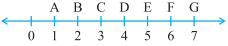### Chapter - 5 Understanding Elementary Shapes

Q
##### Understanding Elementary Shapes

Question:

1. What is the disadvantage in comparing line segments by mere observation?

Solution: The disadvantage in comparing line segments by mere observation is the chance of miscalculation, sometimes we can't judge correctly which line segment is longer by just observing.

2. Why is it better to use a divider than a ruler, while measuring the length of a line segment?

Solution: It is better to use a divider than a ruler because the ruler is thick, while taking a reading there is a change of misread of reading due to thickness.

3. Draw any line segment, say . Take any point C lying in between A and B. Measure the lengths of AB, BC and AC. Is AB = AC + CB?

[Note : If A,B,C are any three points on a line such that AC + CB = AB, then we can be sure that C lies between A and B.]

Solution:Here AB = 10 cm, AC = 4 cm and CB = 6 cm.

Lets check the relation AB = AC + CB

AC + CB = 4 cm + 6 cm  = 10 cm = AB.

Yes, AB = AC + CB.

As, C is the interior point of line segment AB so this relation AB = AC + CB is true for every line segment.

4. If A,B,C are three points on a line such that AB = 5 cm, BC = 3 cm and AC = 8 cm, which one of them lies between the other two?

Solution: Here, AB = 5 cm, BC = 3 cm and AC = 8 cm {Given}

Clearly, the sum of AB and BC is equal to AC.

AB + BC = 5 cm + 3 cm = 8 cm = AC

AB + BC = AC

So, here A and C are the endpoints of the line segment AC and point B lie between points A and C.

5. Verify, whether D is the mid point of .Solution: In the figure shown, we can see

AD = 4 - 1 = 3 ----(1)

DG = AG - AD = 7 - 4 = 3 -----(2)

From (1) and (2),

Hence, D is the mid point of .

6. If B is the mid point of and C is the mid point of , where A,B,C,D lie on a straight line, say why AB = CD?

Solution:It is given that B is a mid point of .

So, by this AB = BC ----(1)

Now, it is given that C is a mid point of .

So, BC = CD -----(2)

From (1) and (2), we get

AB = CD.

7. Draw five triangles and measure their sides. Check in each case, if the sum of the lengths of any two sides is always less than the third side.

Solution: (a)In ABC,

AB = 5 cm, BC = 6 cm and AC = 5 cm

AB + BC = 5 cm + 6 cm = 11 cm

11 cm > 5 cm

AB + BC > AC

Hence, the sum of the lengths of any two sides is greater than the third side.

(b)In MNO,

MN = 8 cm, ON = 9 cm and OM = 5 cm

MN + OM = 8 cm + 5 cm = 13 cm

13 cm > 9 cm

MN + OM > ON

Hence, the sum of the lengths of any two sides is greater than the third side.

(c)In XYZ,

XY = 4 cm, YZ = 6 cm and XZ = 3 cm

XY + XZ = 4 cm + 3 cm = 7 cm

7 cm > 6 cm

XY + XZ > YZ

Hence, the sum of the lengths of any two sides is greater than the third side.

(d)In PQR,

PR = 5 cm, RQ = 4 cm and PQ = 3 cm

RQ + PQ = 4 cm + 3 cm = 7 cm

7 cm > 5 cm

RQ + PQ > PR

Hence, the sum of the lengths of any two sides is greater than the third side.

(e)In DEF,

DE = 5 cm, EF = 6 cm and DF = 8 cm

DE + EF = 5 cm + 6 cm = 11 cm

11 cm > 8 cm

DE + EF > DF

Hence, the sum of the lengths of any two sides is greater than the third side.

Therefore, from all five cases, we can conclude that the sum of the lengths of any two sides is always greater than the third side.